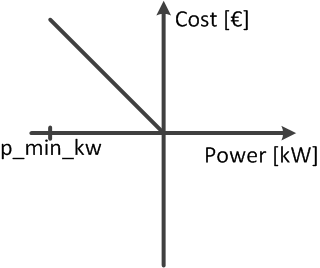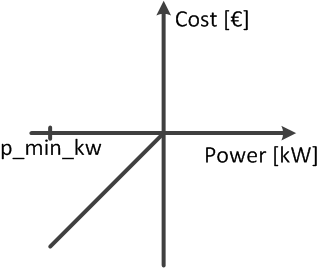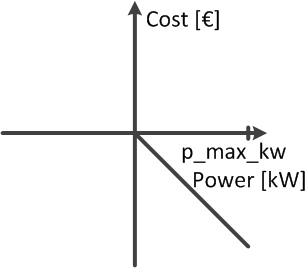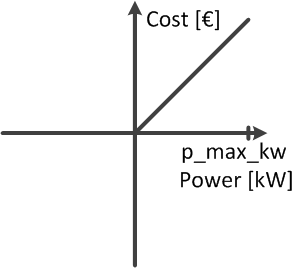# Optimisation problem¶

The equation describes the basic formulation of the optimal power flow problem. The pandapower optimal power flow can be constrained by either, AC and DC loadflow equations. The branch constraints represent the maximum apparent power loading of transformers and the maximum line current loadings. The bus constraints can contain maximum and minimum voltage magnitude and angle. For the external grid, generators, loads, DC lines and static generators, the maximum and minimum active resp. reactive power can be considered as operational constraints for the optimal power flow. The constraints are defined element wise in the respective element tables.

$\begin{split}& min & \sum_{i \ \epsilon \ gen, sgen, load, extgrid }{f_{i}(P_i)} \\ & subject \ to \\ & & Loadflow \ equations \\ & & branch \ constraints \\ & & bus \ constraints \\ & & operational \ power \ constraints \\\end{split}$

Generator Flexibilities / Operational power constraints

The active and reactive power generation of generators, loads, dc lines and static generators can be defined as a flexibility for the OPF.

 Constraint Defined in $$P_{min,i} \leq P_{g} \leq P_{max,g}, g \ \epsilon \ gen$$ net.gen.min_p_kw / net.gen.max_p_kw $$Q_{min,g} \leq Q_{g} \leq Q_{max,g}, g \ \epsilon \ gen$$ net.gen.min_q_kvar / net.gen.max_q_kvar $$P_{min,sg} \leq P_{sg} \leq P_{max,sg}, sg \ \epsilon \ sgen$$ net.sgen.min_p_kw / net.sgen.max_p_kw $$Q_{min,sg} \leq Q_{sg} \leq Q_{max,sg}, sg \ \epsilon \ sgen$$ net.sgen.min_q_kvar / net.sgen.max_q_kvar $$P_{max,g}, g \ \epsilon \ dcline$$ net.dcline.max_p_kw $$Q_{min,g} \leq Q_{g} \leq Q_{max,g}, g \ \epsilon \ dcline$$ net.dcline.min_q_from_kvar / net.dcline.max_q_from_kvar / net.dcline.min_q_to_kvar / net.dcline.max_q_to_kvar $$P_{min,eg} \leq P_{eg} \leq P_{max,eg}, eg \ \epsilon \ ext_grid$$ net.ext_grid.min_p_kw / net.ext_grid.max_p_kw $$Q_{min,eg} \leq Q_{eg} \leq Q_{max,eg}, eg \ \epsilon \ ext_grid$$ net.ext_grid.min_q_kvar / net.ext_grid.max_q_kvar $$P_{min,ld} \leq P_{ld} \leq P_{max,ld}, ld \ \epsilon \ load$$ net.sgen.min_p_kw / net.sgen.max_p_kw $$Q_{min,ld} \leq Q_{ld} \leq Q_{max,ld}, ld \ \epsilon \ load$$ net.sgen.min_q_kvar / net.sgen.max_q_kvar

Note

Defining operational constraints is indispensable for the OPF, it will not start if contraints are not defined.

Network Constraints

The network constraints contain constraints for bus voltages and branch flows:

 Constraint Defined in $$V_{min,j} \leq V_{g,i} \leq V_{min,i}, j \ \epsilon \ bus$$ net.bus.min_vm_pu / net.bus.max_vm_pu $$L_{k} \leq L_{max,k}, k \ \epsilon \ trafo$$ net.trafo.max_loading_percent $$L_{l} \leq L_{max,l}, l \ \epsilon \ line$$ net.line.max_loading_percent $$L_{l} \leq L_{max,l}, l \ \epsilon \ trafo_{3w}$$ net.trafo3w.max_loading_percent

The defaults are 100% loading for branch elements and +-0.1 p.u. for bus voltages.

## Cost functions¶

The cost function is specified element wise and is organized in tables as well, which makes the parametrization user friendly. There are two options formulating a cost function for each element: A piecewise linear function with $n$ data points.

$\begin{split}f_{pwl}(p) = f_{\alpha} +(p-p_{\alpha}) \frac{f_{\alpha + 1}-f_{\alpha}}{p_{\alpha + 1}-p_{\alpha}} \ , \ (p_{\alpha},f_{\alpha}) \ =\begin{cases} (p_{0},f_{0}) \ , \ & p_{0} < p <p_{1}) \\ ...\\ (p_{n-1},f_{n-1}) \ , & \ p_{n-1} < p <p_{n}) \end{cases} \\ \\ f_{pwl}(q) = f_{1} +(q-q_{1}) \frac{f_{2}-f_{1}}{q_{2}-q_{1}}\end{split}$

Piecewise linear cost functions can be specified using create_piecewise_linear_costs():

pandapower.create_piecewise_linear_cost(net, element, element_type, data_points, type='p', index=None)
Creates an entry for piecewise linear costs for an element. The currently supported elements are
• Generator
• External Grid
• Static Generator
• Dcline
• Storage
INPUT:

element (int) - ID of the element in the respective element table

element_type (string) - Type of element [“gen”, “sgen”, “ext_grid”, “load”, “dcline”, “storage”] are possible

data_points - (numpy array) Numpy array containing n data points (see example)

OPTIONAL:

type - (string) - Type of cost [“p”, “q”] are allowed

index (int, index) - Force a specified ID if it is available. If None, the index one higher than the highest already existing index is selected.

OUTPUT:
index (int) - The unique ID of created cost entry
EXAMPLE:
create_piecewise_linear_cost(net, 0, “load”, np.array([[0, 0], [75, 50], [150, 100]]))
NOTE:
• costs for reactive power can only be quadratic, linear or constant. No higher grades supported.
• costs for storages are positive per definition (similar to sgen costs)

The other option is to formulate a n-polynomial cost function:

$\begin{split}f_{pol}(p) = c_n p^n + ... + c_1 p + c_0 \\ f_{pol}(q) = c_2 q^2 + c_1 q + c_0\end{split}$

Polynomial cost functions can be speciefied using create_polynomial_cost():

pandapower.create_polynomial_cost(net, element, element_type, coefficients, type='p', index=None)
Creates an entry for polynomial costs for an element. The currently supported elements are
• Generator
• External Grid
• Static Generator
• Dcline
• Storage
INPUT:

element (int) - ID of the element in the respective element table

element_type (string) - Type of element [“gen”, “sgen”, “ext_grid”, “load”, “dcline”, “storage”] are possible

data_points - (numpy array) Numpy array containing n cost coefficients, starting with highest order (see example)

**type ** -“p” or “q”

OPTIONAL:

type - (string) - Type of cost [“p”, “q”] are allowed

index (int, None) - Force a specified ID if it is available. If None, the index one higher than the highest already existing index is selected.

OUTPUT:
index (int) - The unique ID of created cost entry
EXAMPLE:
create_polynomial_cost(net, 0, “gen”, np.array([0, 1, 0]))
NOTE:
• costs for storages are positive per definition (similar to sgen costs)

Note

Please note, that polynomial costs for reactive power can only be quadratic, linear or constant. Piecewise linear cost funcions for reactive power are not working at the moment with 2 segments or more. Loads can only have 2 data points in their piecewise linear cost function for active power.

Active and reactive power costs are calculted seperately. The costs of all types are summed up to determine the overall costs for a grid state.

## Visualization of cost functions¶

Minimizing Generation

The most common optimization goal is the minimization of the overall generator feed in. The according cost function would be formulated like this:

pp.create_polynomial_cost(net, 0, 'sgen', np.array([-1, 0]))
pp.create_polynomial_cost(net, 0, 'gen', np.array([-1, 0]))
pp.create_polynomial_cost(net, 0, 'ext_grid', np.array([-1, 0]))
pp.create_piecewise_linear_cost(net, 0, "sgen", np.array([[net.sgen.min_p_kw.at, 1000], [0, 0]]))
pp.create_piecewise_linear_cost(net, 0, "gen", np.array([[net.gen.min_p_kw.at, 1000], [0, 0]]))
pp.create_piecewise_linear_cost(net, 0, "ext_grid", np.array([[-1e9, 1e9], [1e9, -1e9]]))


It is a straight with a negative slope, so that it has the highest cost value at p_min_kw and is zero when the feed in is zero:Maximizing generation

This cost function may be used, when the curtailment of renewables should be minimized, which at the same time means that the feed in of those renewables should be maximized. This can be realized by the following cost function definitions:

pp.create_polynomial_cost(net, 0, 'sgen', np.array([1, 0]))
pp.create_polynomial_cost(net, 0, 'gen', np.array([1, 0]))
pp.create_piecewise_linear_cost(net, 0, "sgen", np.array([[net.sgen.min_p_kw.at, -1000], [0, 0]]))
pp.create_piecewise_linear_cost(net, 0, "gen", np.array([[net.gen.min_p_kw.at, -1000], [0, 0]]))
pp.create_piecewise_linear_cost(net, 0, "ext_grid", np.array([[-1e9, -1e9], [1e9, 1e9]]))


It is a straight with a positive slope, so that the cost is zero at p_min_kw and is at its maximum when the generation equals zero.In case that the load should be maximized, the cost function could be defined like this:

pp.create_polynomial_cost(net, 0, 'load', np.array([-1, 0]))
pp.create_polynomial_cost(net, 0, 'storage', np.array([-1, 0]))
pp.create_piecewise_linear_cost(net, 0, "sgen", np.array([[0, 0], [net.load.max_p_kw.at, -1000]]))
pp.create_piecewise_linear_cost(net, 0, "gen", np.array([[net.storage.min_p_kw.at, 1000], [net.storage.max_p_kw.at, -1000]]))In case that the load should be minimized, the cost function could be defined like this:

pp.create_polynomial_cost(net, 0, 'load', np.array([1, 0]))
pp.create_polynomial_cost(net, 0, 'storage', np.array([1, 0]))
pp.create_piecewise_linear_cost(net, 0, "sgen", np.array([[0, 0], [net.load.max_p_kw.at, 1000]]))
pp.create_piecewise_linear_cost(net, 0, "gen", np.array([[net.storage.min_p_kw.at, -1000], [net.storage.max_p_kw.at, 1000]]))DC line behaviour

Please note, that the costs of the DC line transmission are always related to the power at the from_bus!